# Get minimum values in rows or columns with their index position in Pandas-Dataframe

• Difficulty Level : Medium
• Last Updated : 02 Jul, 2020

Let’s discuss how to find minimum values in rows & columns of a Dataframe and also their index position.

a) Find the minimum value among rows and columns :

Attention geek! Strengthen your foundations with the Python Programming Foundation Course and learn the basics.

To begin with, your interview preparations Enhance your Data Structures concepts with the Python DS Course. And to begin with your Machine Learning Journey, join the Machine Learning - Basic Level Course

Dataframe.min() : This function returns the minimum of the values in the given object. If the input is a series, the method will return a scalar which will be the minimum of the values in the series. If the input is a dataframe, then the method will return a series with a minimum of values over the specified axis in the dataframe. By default, the axis is the index axis.

1) Get minimum values of every column :
Use min() function to find the minimum value over the index axis.

Code :

 # import pandas libraryimport pandas as pd  # list of Tuplesdata = [          (20, 16, 23),          (30, None, 11),          (40, 34, 11),          (50, 35, None),          (60, 40, 13)        ]  # creating a DataFrame objectdf = pd.DataFrame(data, index = ['a', 'b', 'c', 'd', 'e'],                     columns = ['x', 'y', 'z'])  # getting a series object containing # minimum value from each column# of given dataframeminvalue_series = df.min()  minvalue_series

Output: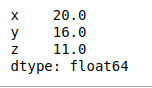2) Get minimum values of every row :
Use min() function on a dataframe with ‘axis = 1’ attribute to find the minimum value over the row axis.

Code :

 # import pandas libraryimport pandas as pd  # list of Tuplesdata = [          (20, 16, 23),          (30, None, 11),          (40, 34, 11),          (50, 35, None),          (60, 40, 13)        ]  # creating a DataFrame objectdf = pd.DataFrame(data, index = ['a', 'b', 'c', 'd', 'e'],                     columns = ['x', 'y', 'z'])  # getting a series object containing # minimum value from each row# of given dataframeminvalue_series = df.min(axis = 1)  minvalue_series

Output: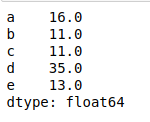3) Get minimum values of every column without skipping None Value :
Use min() function on a dataframe which has Na value with ‘skipna = False’ attribute to find the minimum value over the column axis.

Code :

 # import pandas libraryimport pandas as pd  # list of Tuplesdata = [          (20, 16, 23),          (30, None, 11),          (40, 34, 11),          (50, 35, None),          (60, 40, 13)        ]  # creating a DataFrame objectdf = pd.DataFrame(data, index = ['a', 'b', 'c', 'd', 'e'],                     columns = ['x', 'y', 'z'])  # getting a series object containing # minimum value from each column# of given dataframe without# skipping None valueminvalue_series = df.min(skipna = False)  minvalue_series

Output: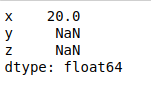4) Get minimum value of a single column :
Use min() function on a series to find the minimum value in the series.

Code :

 # import pandas libraryimport pandas as pd  # list of Tuplesdata = [          (20, 16, 23),          (30, None, 11),          (40, 34, 11),          (50, 35, None),          (60, 40, 13)        ]  # creating a DataFrame objectdf = pd.DataFrame(data, index = ['a', 'b', 'c', 'd', 'e'],                     columns = ['x', 'y', 'z'])  # getting a minimum value# from column 'x'minvalue = df['x'].min()  minvalue

Output:

20

b) Get row index label or position of minimum values among rows and columns :

Dataframe.idxmin() : This function returns index of first occurrence of minimum over requested axis. While finding the index of the minimum value across any index, all NA/null values are excluded.

1) Get row index label of minimum value in every column :
Use idxmin() function to find the index/label of the minimum value along the index axis.

Code :

 # import pandas libraryimport pandas as pd  # list of Tuplesdata = [          (20, 16, 23),          (30, None, 11),          (40, 34, 11),          (50, 35, None),          (60, 40, 13)        ]  # creating a DataFrame objectdf = pd.DataFrame(data, index = ['a', 'b', 'c', 'd', 'e'],                     columns = ['x', 'y', 'z'])  # get the index position\label of# minimum values in every columnminvalueIndexLabel = df.idxmin()  minvalueIndexLabel

Output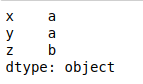2) Get Column names of minimum value in every row :
Use idxmin() function with ‘axis = 1’ attribute to find the index/label of the minimum value along the column axis.

Code :

 # import pandas libraryimport pandas as pd  # list of Tuplesdata = [          (20, 16, 23),          (30, None, 11),          (40, 34, 11),          (50, 35, None),          (60, 40, 13)        ]  # creating a DataFrame objectdf = pd.DataFrame(data, index = ['a', 'b', 'c', 'd', 'e'],                     columns = ['x', 'y', 'z'])  # get the index position\label of# minimum values in every rowminvalueIndexLabel = df.idxmin(axis = 1)  minvalueIndexLabel

Output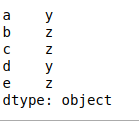My Personal Notes arrow_drop_up